• Sharon (not verified) 11 years 10 weeks ago

What is a "smart exhaust"?

• 11 years 10 weeks ago

This is a nice comment of yours, and it does seem to me that the best thing to do Tricki-wise is what you more or less suggest. That is, in this article one could say something like, "It is not hard to prove that the numbers,and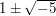cannot be factorized any further" and then say "For a proof, see ..." Then there could be a separate article on this norm trick. Or perhaps the norm trick could be thought of as an example of the more general trick, which actually came up in a discussion about proving facts about groups, of finding a homomorphism to some structure that's simpler to analyse.

• Michael Lugo (not verified) 11 years 10 weeks ago

Is it clear to the intended reader that none of those numbers can be factored any further? Of course one does something like trial division, but it's not obvious what "something like trial division" is.

One possible proof that 2, 3, 1 + \sqrt{-5}, 1 - \sqrt{-5} can't be factored any further is as follows. Let N(a+b\sqrt{-5}) = a^2 + 5b^2 (I use N because this is a "norm".) This norm is multiplicative, since it's the square of the complex absolute value. Then if, say, there exist non-unit elements of Z(\sqrt{-5}), a + b\sqrt{-5} and c + d\sqrt{-5}, such that

(a + b \sqrt{-5}) (c + d \sqrt{-5}) = 3

then they have norms either 1 and 9 or 3 and 3, respectively. There are no elements of the ring with norm 3, and the only elements of norm 1 are units. Similar proofs work for 2, 1 + \sqrt{-5}, 1 - \sqrt{-5} – in general, if an element of Z(\sqrt{-5}) can be factored, then its norm can be factored, and the factorization of a + b\sqrt{-5} will be a "lifting" of the factorization of the norm a^2 + 5b^2.

("Take norms" seems like its own trick, and perhaps this is better placed in an article on that trick.)

• 11 years 10 weeks ago

Closely related is Péron's expression solving the harmonic Dirichlet problem, as an infimum of sup-harmonic dominators.

• Louis (not verified) 11 years 10 weeks ago Greedy algorithms

I'm not really sure about the etiquette for this site, so I hope this comment isn't too low-level.

In computer science, at least, pretty much the first example of greedy algorithms is for the problem of finding a minimum weight spanning tree in a graph (and then the generalization to the characterization of matroids by greedy algorithms).

Also, sometimes when greedy algorithms don't work optimally, you can still get something useful, like finding a linear-sized independent set in a planar graph or approximating set cover. (There are many examples of this nature.)

• 11 years 10 weeks ago

Oh yes... "tricks" wiki... yeah, it came up in a complex/harmonic analysis course I was taking, but the context... remains elusive.

• Anonymous (not verified) 11 years 10 weeks ago

This looks nice, but do you plan to give some examples of problems where this trick is useful? It would be a big help.

• Danny Calegari (not verified) 11 years 11 weeks ago

I have a couple of posts on my blog (http://lamington.wordpress.com) with lots of examples of ways to show that a group is finite/infinite, probably too many/too specialized for the tricki. You are welcome to mine these posts for anything you think is relevant.

• Anonymous (not verified) 11 years 11 weeks ago

• Anonymous (not verified) 11 years 12 weeks ago

In fact, nowhere dense sets can not only be uncountable, they can can positive measure. See the wikipedia page on the Smith-Volterra-Cantor set. This set is a good source for counterexamples.

• 11 years 12 weeks ago

Yes. The preamble to the theorem states that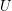is finite dimensional and irreducible. If you want to makes these conditions explicit in the statement of the theorem, feel free to add them.

• 11 years 12 weeks ago

Corrected, thanks!

• Mike Steele (not verified) 11 years 12 weeks ago

Some of the Latex is not rendered appropirately.

• 11 years 12 weeks ago Basic examples of groups

Articles along those kinds of lines sound like a great idea to me.

• 11 years 12 weeks ago How to use martingales

Thanks – I've corrected it. I've tended to deal with martingales, such as the one in this permutations example, where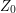is constant, which is why I wrote what I wrote.

• 11 years 12 weeks ago How to use martingales

The article says

"Then the martingale condition is that the expectation of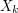given the values of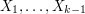is always 0."

I see that the martingale condition implies this, but is it true that the martingale condition "is" this? This condition can be satisfied without the sequence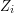being a martingale.

Indeed, suppose A, B are independent random variables with mean zero. Define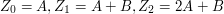. Clearly,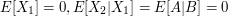, so the above condition holds. On the other this is not a martingale: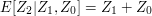which may not equal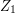.

• Gabe Cunningham (not verified) 11 years 13 weeks ago Basic examples of groups

In the spirit of this page, would it be useful/desirable to have minimal examples of groups with certain properties (or failing to have certain properties?) Another similar idea would be to make a table using several basic group properties (e.g., abelian, simple, finite, torsion) and to give an example group or family of groups for each possible combination of properties. Perhaps such a table would be better served as part of a separate article detailing various nice properties of groups, with crosslinks to and from here. I'd be happy to start such a page if there is interest.

• 11 years 13 weeks ago How to use fixed point theorems

I would like to mention that this kind of results, in particular Kakutani's fixed point theorem, can be used to produce invariant subspaces for operators on Banach spaces. Added later: where I said Kakutani, I meant to say Ky Fan.

• 11 years 13 weeks ago How to use fixed point theorems

An application of this result is Lomonosov's remarkable theorem about the existence of invariant subspaces for an operator on a Banach space that commutes with a non zero compact operator. It would be nice to write an article on this. Also, it could be linked from other articles like

analysis > Operator theory

How to prove the existence of > Invariant subspaces

• 11 years 13 weeks ago How to use fixed point theorems

A nice application of this principle is Picard's theorem about the existence of solutions to an initial value problem for a first order, ordinary differential equation. The map involved is highly non linear and the solution cannot be written down explicitily as in example 2. The idea is to convert the differential equation into an integral equation. The solution is then a fixed point for the operator that rules the integral equation. I just realized that there is a reference to this kind of trick at the end of the quick description above.

• Anonymous (not verified) 11 years 13 weeks ago

Does Theorem 1 require that U is simple? The proof (use of Schur's lemma) seems to assume this.

• Anonymous (not verified) 11 years 13 weeks ago Applying the probabilistic method

There might be a mistake

According to the line "There is also a simple but revolutionary argument of Erd?s that proves that is at least (1+0(1))k 2^k /e sqr(2). "

If (1+0(1))k 2^k /e sqr(2) is the right estimate, then there might be a mistake in the straightforward computation part in the article. For the first estimate of n, it might miss the constant k and without sqr(2). Thus, the final estimate of n is missing a k and the sqr(2)is justified from taking the -1/2 power of 2^[(k-1)/2].

• Toni (not verified) 11 years 13 weeks ago Enumerative combinatorics front page

Does it apply to texas poker?

• 11 years 13 weeks ago Induction front page

"The generalized induction is harder because we don't have a nice indexing set like the natural numbers. Is there a way of doing induction without the crutch of an indexing set? Yes there is: you look for a minimal counterexample."